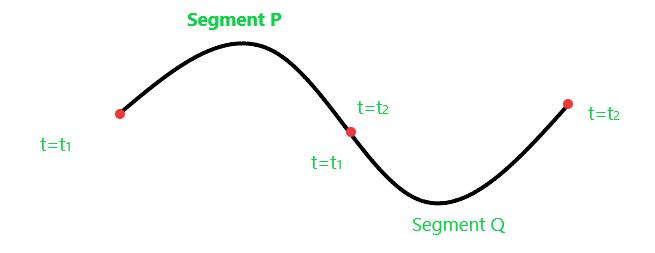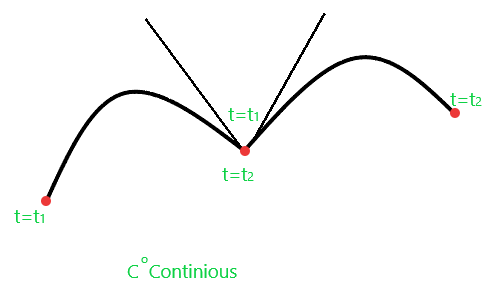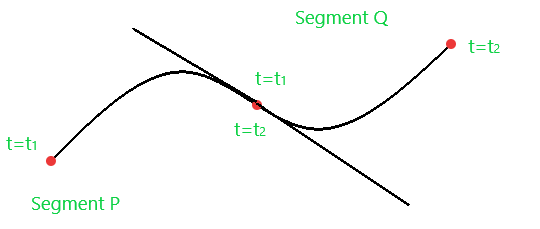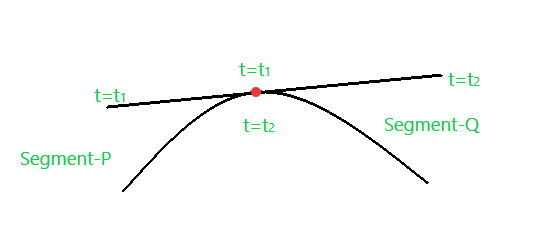# Parametric & Geometric Continuity of Curves in Computer Graphics

• Last Updated : 27 Jan, 2022

Prerequisite: B-spline curve, Bezier curve

The continuity condition represents that how smoothly a curve transit from one curve segment to another segment. Consider you are given a curve as shown below:There are three kinds of Parametric continuities that exist:

(a) Zero-order parametric continuity(_C0 ) : A curve is said to be zero-order parametric continuous if both segments of the curve intersect at one endpoint.

`                                        P(t2) =  Q(t1)`(b) First-order parametric continuity(C1) : A curve is said to be first-order parametric continuous if it is Co Continuous and the first-order derivative of segment P at t=t is equal to the first-order derivative of segment Q at t=t1. Such kinds of curves have the same tangent line at the intersection point.

`                                     P'(t2) = Q'(t1)`(c) Second-order parametric continuity(C2) : A curve is said to be second-order parametric continuous if it is Co and C1 Continuous and the second-order derivative of the segment P at t=t1 is equal to the second-order derivative of segment Q at t=t2

`                                    P''(t2) = Q''(t1)`Geometric Continuity : It is an alternate method for joining two curve segments, where it requires the parametric derivation of both segments which are proportional to each other rather than equal to each other.

(a) Zero-order geometric continuity(Go) : It is similar to the zero-order parametric curve continuity condition.

`                                         P(t2) =  Q(t1)`

(b) First-order geometric continuity(G1) : The junction point between two points is said to be G1 continuous if the coordinate of both curve segments is G0 continuous and following the below condition:

`                                 P'(t2) =  k * Q'(t1)  for all x, y, z and k > 0.`

(c) Second-order geometric continuity(G2) : The junction point between two points is said to be G2 continuous if the coordinate of both curve segments is G1 continuous and following the below condition:

`                                P''(t2) =  k * Q''(t1)  for all x, y, z and k > 0.`
My Personal Notes arrow_drop_up